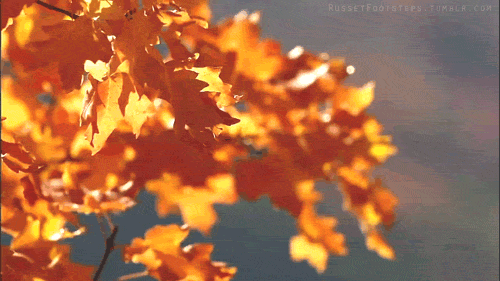# Curiosity-driven Exploration by Self-supervised Prediction

## TL;DR

Curiosity = the error in agent’s ability to predict the consequence of its own actions in a feature space learned by a self-supervised inverse dynamics model.

## Introduction

Objective of Reinforcement Learning is to find an agent parameterised by $$\theta$$ that maximise expected the sum of rewards over the policy trajectory.

Mathematically, it can be shown as:

$\theta^* = arg \max_{\theta} E_{\tau \sim p_{\theta}(\tau)} [\sum_t r_t]$

The reinforcement learning framework is usually represented as Markov Decision Process which is tuple of $$\{S,A,T,r\}$$, where:

S: State Space A: Action Space T: Transition Operator r: rewards

And this can pictured as:

So, clearly the only learning signal we are providing to the agent is through the rewards.

### Problem with Rewards

• Extrinsic rewards are extremely sparse, or absent completely in more realistic scenarios
• Extrinsic Rewards are usually hand-made
• Reward Shaping in itself is a very tedious & complex task
• Only learning signal for RL agents is from reward signals

## A Solution: Add more rewards?

### Intrinsic Motiviation

We want to make the reward structure more dense so that it’s easier for agents to learn. So, we want to create some extra rewards using all the information availbale to us.

Using all the current information we have, we want to motivate the agent to expore more, i.e. generate rewards for unexplored states. Mathematically,

$r_t = r_t^i + r_t^e \\ \theta^* = arg \max_{\theta} E_{\tau \sim p_{\theta}(\tau)} [\sum_t r_t]$

There are 2 broad classes of techniques to achieve this:

1. encourage the agent to explore “novel” states (Bellemare et al., 2016; Lopes et al., 2012; Poupart et al., 2006) or,
2. encourage the agent to perform actions that reduce the error/uncertainty in the agent’s ability to predict the consequence of its own actions (i.e. its knowledge about the environment)

Curiosity falls in the category of “generate an intrinsic reward signal based on how hard it is for the agent to predict the consequences of its own actions” i.e. 2nd Category.

## Prediction Error as Curiosity Reward

### Simple Solution

$\hat{s}_{t+1} = f(s_t, a_t) \\ r^i_t = \eta \lVert \hat{s}_{t+1} - s_{t+1} \rVert_k$

However:

• It’s very hard to predict in raw sensory state like pixels
• Also, it’s unclear if predicting the pixels is the right objective

The underlying problem is that the agent is unaware that some parts of the state space simply cannot be modeled and thus the agent can fall into an artificial curiosity trap and stall its exploration. This is one of the pitfalls of count based exploration, as they try to model a feature space from states directly.

This can better understood with an example such as when the agent tries to the movement of leaves due to a breeze. This is super hard to learn and is also irrelvant for the agent as it does not affect the agent and nor is it controllable by the agent.Trying to predict the movement of leaves is hard, even so in pixel space

Then what to do? Let’s look at the factors contributing to observation space:

1. things that can be controlled by the agent,
2. things that the agent cannot control but that can affect the agent (e.g. a vehicle driven by another agent), and
3. things out of the agent’s control and not affecting the agent (e.g. moving leaves)

A good feature space for curiosity should model (1) and (2) and be unaffected by (3)

Key Insight: we only predict those changes in the environment that could possibly be due to the actions of our agent or affect the agent, and ignore the rest.

* This helps avoid pitfalls of previous work.
* Transform input to feature space learnt by self-supervision
* Since the neural network is only required to predict the action, it has no incentive to represent within its feature embedding space the factors of variation in the environment that don't affect the agent.
* And then use this feature space to learn a forward model


## Self-supervised prediction for exploration

### Inverse Dynamics

This has 2 Sub-modules:

• State Encoder: $$s_t \to \phi (s_t)$$
• Action Predictor: $$\phi (s_{t+1}), \phi (s_t) \to \hat{a_t}$$

This is equivalient to learning function $$g$$ (Inverse Dynamics Model)

$\hat{a}_t = g(s_t, s_{t+1}; \theta_I) \\ \min_{\theta_I} L_I(\hat{a_t}, a_t)$

### Forward Model

$\hat{\phi}(s_{t+1}) = f(\phi(s_t), a_t; \theta_{F}) \\ L_F (\phi(s_{t+1}), \hat{\phi}(s_{t+1})) = \frac{1}{2} \lVert \phi(s_{t+1}) - \hat{\phi}(s_{t+1}) \rVert^2_2 \\ r_t^i = \frac{\eta}{2} \lVert \phi(s_{t+1}) - \hat{\phi}(s_{t+1}) \rVert^2_2$
• Jointly Optimise $$I$$ and $$F$$
• As there is no incentive for this feature space to encode any environmental features that are not influenced by the agent’s actions, our agent will receive no rewards for reaching environmental states that are inherently unpredictable and its exploration strategy will be robust to the presence of distractor objects, changes in illumination, or other nuisance sources of variation in the environment.

## Roles of Curiosity

1. Solve Tasks with Sparse Rewards
2. Explore its env in quest for knowledge, improves as agents gain mroe knwoledge
3. Learn skills for future scenarios

## Experiments

• Diff. levels for train and test
• Trained using visual Inputs
• Current State Stacked with previous 3 states
• Action repeat: 4x VizDoom 6x SuperMario Bros
• No Action repeat during inference

### Environments

1. VizDoom

2. SuperMario Bros (Paquette 2016)

• Trained only on curiosity reward

Main Contribution: designing an intrinsic reward signal based on prediction error of the agent’s knowledge about its environment that scales to high-dimensional continuous state spaces like images, bypasses the hard problem of predicting pixels and is unaffected by the unpredictable aspects of the environment that do not affect the agent.

Tags:

Categories:

Updated: